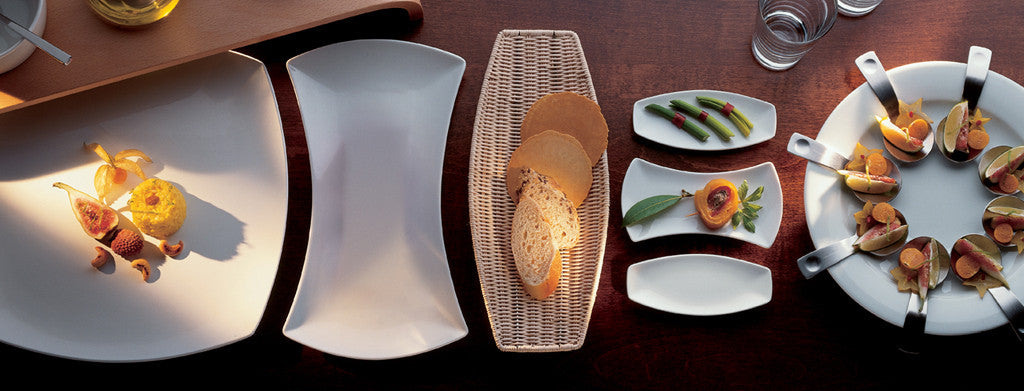Shopping Cart

### Refine

View all

#### Price# Crockery£4.91 each (ex VAT)£7.72 each (ex VAT)£3.72 each (ex VAT)£3.72 each (ex VAT)£5.29 each (ex VAT)£5.29 each (ex VAT)£13.81 each (ex VAT)£9.53 each (ex VAT)£10.85 each (ex VAT)£10.85 each (ex VAT)£3.72 each (ex VAT)£3.72 each (ex VAT)£3.72 each (ex VAT)£5.29 each (ex VAT)£5.29 each (ex VAT)£5.29 each (ex VAT)£5.48 each (ex VAT)£5.48 each (ex VAT)£5.48 each (ex VAT)£5.48 each (ex VAT)£5.48 each (ex VAT)£23.65 each (ex VAT)£23.65 each (ex VAT)£23.65 each (ex VAT)£23.65 each (ex VAT)£23.65 each (ex VAT)£3.09 each (ex VAT)£3.09 each (ex VAT)£3.09 each (ex VAT)£3.09 each (ex VAT)£3.09 each (ex VAT)£3.98 each (ex VAT)£3.98 each (ex VAT)£3.98 each (ex VAT)£3.98 each (ex VAT)£3.98 each (ex VAT)£5.82 each (ex VAT)£5.82 each (ex VAT)£5.82 each (ex VAT)£5.82 each (ex VAT)£5.82 each (ex VAT)£8.61 each (ex VAT)£8.61 each (ex VAT)£8.61 each (ex VAT)£8.61 each (ex VAT)£8.61 each (ex VAT)£13.81 each (ex VAT)£9.53 each (ex VAT)